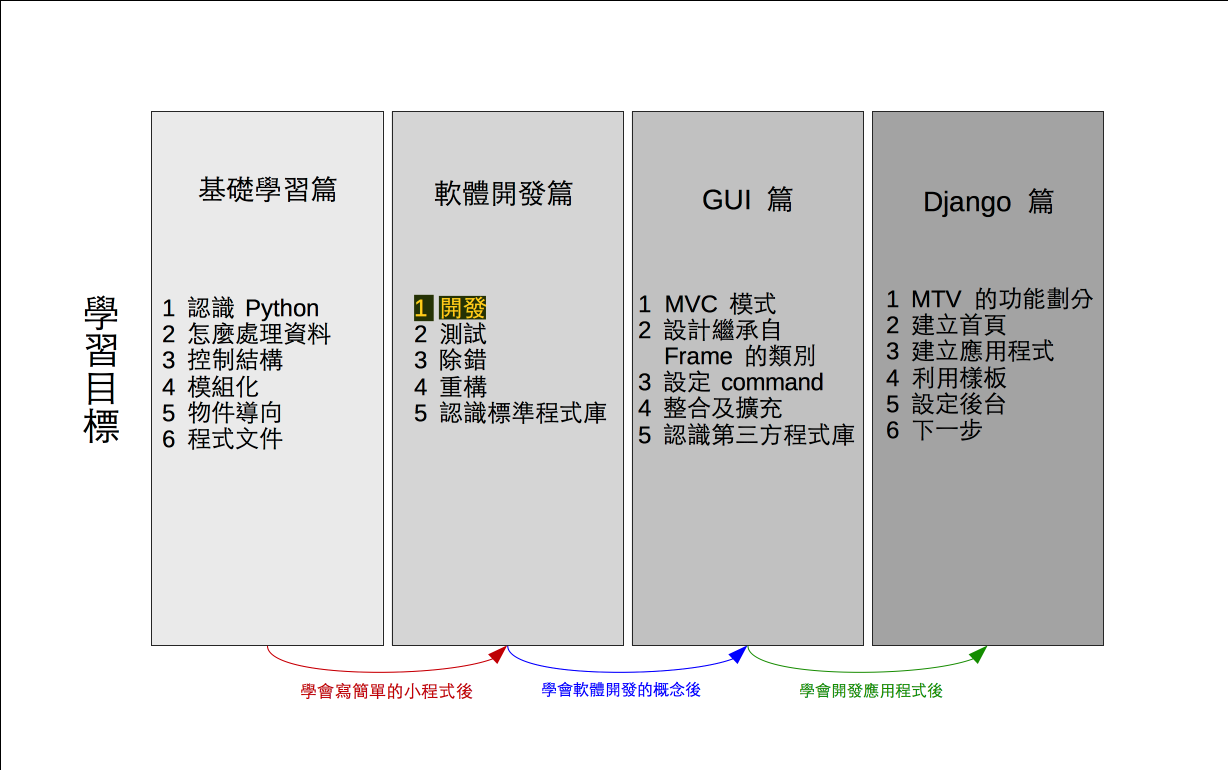# 單元 19 - 解碼

～～學習進度表～～Tbana kw dm wvuur.
×

 0 1 2 3 4 5 6 7 8 9 10 11 12 13 14 15 16 17 18 19 20 21 22 23 24 25 a b c d e f g h i j k l m n o p q r s t w u v x y z

``````# 使用 randint()
import random

# 定義 Encrypt 類別
class Encrypt:
def __init__(self):
self.setcode()

def setcode(self):
# 取得 a 、 b 值
a = 0
b = 0
while a % 2 == 0:
a = random.randint(0, 9)
b = random.randint(0, 9)

# 利用公式建立密碼表
self.code = ""
c = "a"
i = 0
while i < 26:
x = c
y = ord(x) * a + b
m = y % 26
self.code += chr(m + 97)
c = chr(ord(c) + 1)
i += 1
# 第一層迴圈逐一取得每一個字元

def getcode(self):
return self.code

# 編碼的方法
def toEncode(self, str):
# 暫存編碼結果的字串
result = ""

# 利用迴圈走完參數字串的所有字元
for c in str:
# 判斷該字元是否為英文小寫字母
# 若是英文小寫字母就進行編碼轉換
c1 = ord(c) >= 97
c2 = ord(c) <= 122
if c1 and c2:
m = ord(c) - 97
result += self.code[m]
else:
result += c

# 結束回傳編碼過的字串
return result

# 解碼的方法
def toDecode(self, str):
# 暫存解碼結果的字串
result = ""

i = 0
# 第一層迴圈逐一取得每一個字元
while i < len(str):
# 判斷該字元是否為英文小寫字母
# 若是英文小寫字母就進行解碼轉換
i1 = ord(str[i]) >= 97
i2 = ord(str[i]) <= 122
if i1 and i2:
j = 0
# 第二層迴圈尋找該字元在密碼表中
# 的索引值，加上 DIFF 就可轉換
# 回原本的字元
while j < len(self.code):
if str[i] == self.code[j]:
result += chr(j + 97)
j += 1
else:
result += str[i]

i += 1

# 結束回傳解碼過的字串
return result

# 測試部分
if __name__ == '__main__':
e = Encrypt()
print()
print(e.getcode())
s1 = "There is no spoon."
print("Input : " + s1)
s2 = e.toEncode(s1)
print("Encode: " + s2)
s3 = e.toDecode(s2)
print("Decode: " + s3)
print()

#《程式語言教學誌》的範例程式
# http://kaiching.org/
# 檔名：encrypt07.py
# 功能：示範利用 Python 設計 Encrypt 類別
# 作者：張凱慶 */``````

Encrypt 類別到這邊大致已開發完成，最後的測試部分也加入了解碼的相關程式碼

``````# 測試部分
if __name__ == '__main__':
e = Encrypt()
print()
print(e.getcode())
s1 = "There is no spoon."
print("Input : " + s1)
s2 = e.toEncode(s1)
print("Encode: " + s2)
s3 = e.toDecode(s2)
print("Decode: " + s3)
print()``````

 \$ python encrypt07.py wfoxgpyhqzirajsbktcludmven Input : There is no spoon. Encode: Thgtg qc js cbssj. Decode: There is no spoon. \$

1. 解碼與編碼用到相同的轉換表格，因為索引值 0 等於 'a' ，索引值 1 等於 'b' ，餘下可類推。
2. 巢狀迴圈是指迴圈中有其他迴圈，因為用縮排的方式編排程式碼，凹陷下去的地方看起來像鳥巢，故有此名。
3. 解碼利用巢狀迴圈進行，第一層迴圈逐一取得每一個字元，第二層迴圈尋找該字元在密碼表中的索引值。

1. 為什麼編碼跟解碼可以用一樣的轉換表格？
2. 可以不用巢狀迴圈來解碼嗎？
3. 巢狀迴圈可以用一樣名稱的控制變數嗎？

1. 承接上一個單元的猜數字遊戲，將新程式寫在 exercise1901.py 中，修正第一位數可能為 0 的情況。
2. 承上題，將猜數字遊戲改用類別 GuessGame 設計，答案用 answer 屬性儲存，另外設置 timesab 等屬性，把設定以上屬性的程式內容放在 set_game() 方法裡。